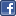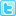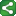Microsoft Excel Resource Center Free Macros, Formulas, Functions, Tutorials, Downloads, Add-Ins & More!
 Monte Carlo Tutorial power4XL Home
 Contents

Building a worksheet-based multiple coin toss Monte Carlo simulationShareThis

Now that we'll take the simple coin toss simulation a step further.

Suppose we want to know:
1. What is the probability of getting "heads" on seven out of ten tosses, and
2. What is the probability of getting "heads" on at least  seven out of ten tosses?

As in the case of the single coin toss, we can solve the problem mathematically rather than through simulation.  In ten coin tosses there are exactly 2^10, or 1024, possible outcomes of heads-tails combinations.  Of these, exactly 120 have seven "heads", so the probability of getting exactly seven "heads" in ten coin tosses is 120/1024, or 11.7%.  Furthermore, 176 of the 1024 combinations have 7, 8, 9, or 10 heads, so the probability of getting heads on at least seven tosses is 176/1024, or 17.2%.

For this example, we use the same formula to simulate a single toss that we used in the previous example.  In each of cells A15 through J15, enter the formula "=RANDBETWEEN(0,1)" as illustrated below.

In cell L15, sum the results of columns A through J by entering "=SUM(A15:J15)" as shown above.  This represents the number of "heads" that came up in ten random tosses of a coin.

Now make 5,000 copies of row 15 in the rows below it.  Thus we have a Monte Carlo worksheet-based simulation representing 5,000 iterations of tossing a coin ten times.

To analyze the results, we make use of the COUNT() and COUNTIF() functions.  As illustrated below, in cell K5 we enter the formula "=COUNT(L15:L5014)" to return the exact number of iterations in the model.  In K6 we count the number of iterations which returned exactly seven heads by entering the formula "=COUNTIF(L15:L5014,7)".  Cell L6 returns the percent of all iterations that resulted in seven heads ("=K6/K5)", and in this particular instance the outcome was 11.2% - reasonably close to the expected statistical outcome of 11.7%.

Cell K7 counts the number of iterations which returned AT LEAST seven heads.  The formula in that cell is "=COUNTIF(L15:L5014,">6")".  L7 shows that result as a percent of all iterations, and again our outcome of 16.6% is reasonably close to the statistical probability of 17.2%.

Copyright notice:  This site and all content, including computer code and spreadsheet examples, are copyright 2006 by Fritz Dooley.  License is granted for individual users to download examples and to copy code directly into user's spreadsheets and Visual Basic for Applications files.  Users may not redistribute code in any way.  Providing hyperlinks to this web site is encouraged, but posting code and examples on other web sites is expressly forbidden.  "Microsoft" is a registered trademark of Microsoft Corporation.   Neither this web site nor Fritz Dooley is affiliated with Microsoft Corporation.